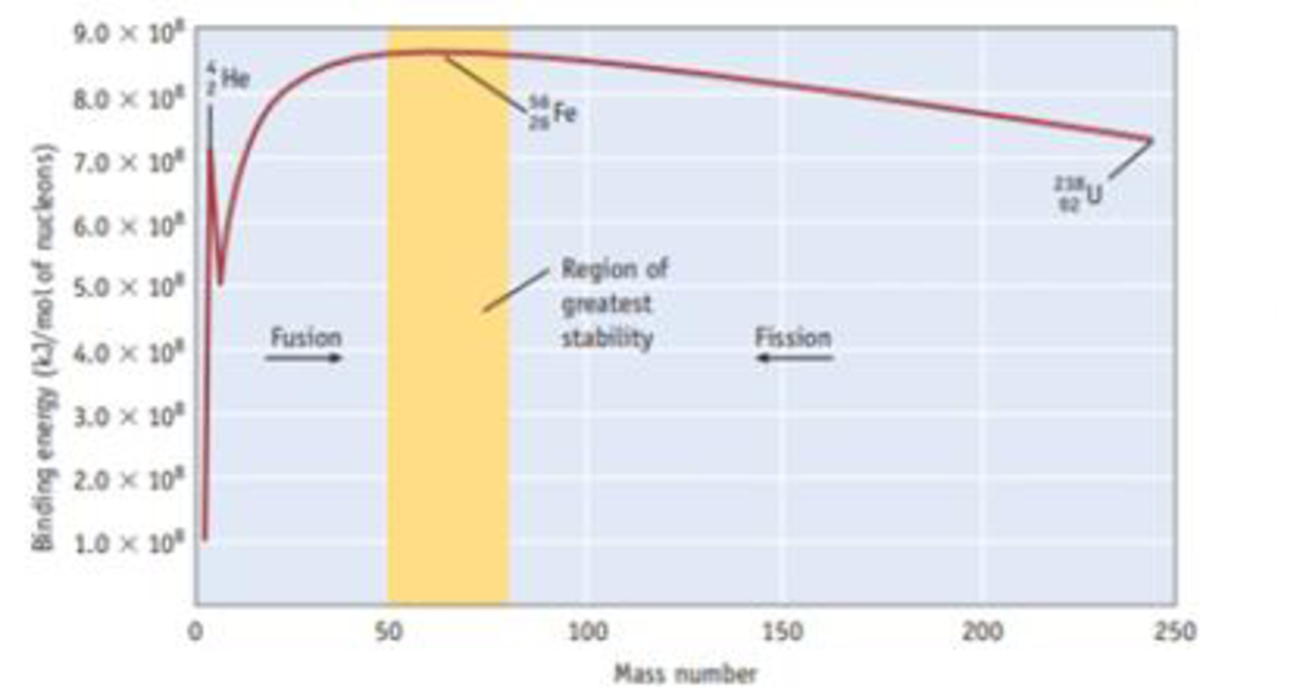Chapter 25, Problem 36PS

Chapter
Section
Textbook Problem

Calculate the binding energy per mole of nucleons for iron-56. Masses needed for this calculation (in g/mol) are H 1 1 = 1.00783, n 0 1 = 1.00867, and F 26 56 e = 55.9349. Compare the result of your calculation to the value for iron-56 in the graph in Figure 25.4.Figure 25.4 Relative stability of nuclei. Binding energy per nucleon for the most stable isotope of elements between hydrogen and uranium is plotted as a function of mass number. (Fission and fusion are discussed in Sectionc25.6.)

Interpretation Introduction

Interpretation:

Binding energy per nucleon of Iron-56 has to be calculated and compared with the given figure.

Concept introduction:

Binding energy is the energy required to separate the nucleus of an atom into proton and neutron. Binding energy is given by Eb=Δm×(c)2, where Δm is the mass defect and c is the velocity of light =3×108m/sec-1

Binding energy per nucleon =TotalbindingenergyofnucleusNumberofnucleonsinthenucleus

Explanation

The given masses are,

H11=1.00783(mass of protons)n01=1.00867(massofneutrons)F2656e=55.9349(ActualmassofFe)

An iron-56 nucleus has 26 protons and (56-26) =30 neutrons. The total mass is thus,

26×1.00728+30×1.00867=56.44938amu.

The total mass of the iron nucleus is =56.44938

On calculating the mass defect,

Δm=M-m.

Here,

M=Total mass (number of protons-number of neutrons)

m=Actual mass (number of neutrons-number of protons)

Δm= Total mass-Actual mass

=56.44938-55.9349=0.5144amu=0.514g/mol= 0.514×10-3kg/mol

Binding energy

=0.514×10-3kg/mol×(3×108m/s)2=4

Still sussing out bartleby?

Check out a sample textbook solution.

See a sample solution

The Solution to Your Study Problems

Bartleby provides explanations to thousands of textbook problems written by our experts, many with advanced degrees!

Get Started